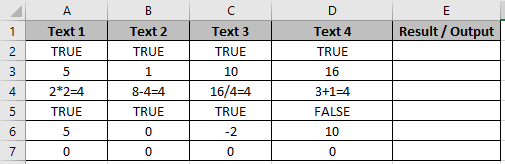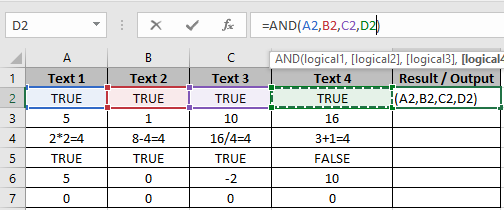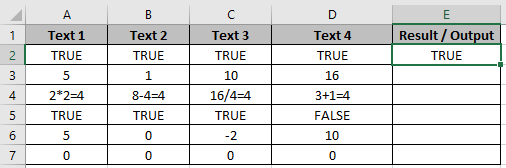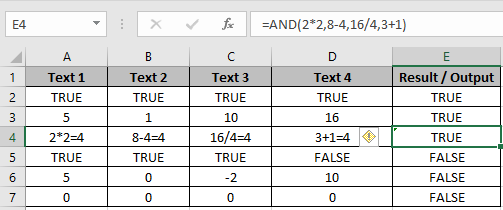# How to use AND function in Microsoft Excel

In this article, we will learn how to use OR function in Excel.

AND function in Excel runs a basic logical function to test two values or statments. OR function checks if any of the condition in formula is False, returns False and it returns True only if all condition or statements are True.

Syntax:

=AND(logical1, [logical2], [logical3], …)

OR function can be used via many ways.
Let’s understand them one by one
Here we have few Examples to test multiple conditions and statements of AND function.Use the formula in E2 cell.

=AND(A2,B2,C2,D2)Press EnterIt returns True as all the statements return True.

There are some default AND Excel statements

1. All numbers except 0 are considered True.
2. 0 is considered as False.
3. True is True and False is False.

Apply the formula in other cells using Ctrl + D taking the first applied cells till the cell you wish to copy formula.In the E2 cell, we applied the formula

=AND(2*2,8-4,16/4,3+1)

We test the 4 conditions in this AND formula. All conditions returns 4 which is considered True is Excel.

If all conditions returns positive numbers and any of it returns 0 then the formula will return False.

You can check less than or greater than condition on numbers. OR function can also be used along with many function like IF, AND and many more.

Hope you understood how to use OR function in Excel. Explore more articles on logic_test functions here. Feel free to state your queries in the comment box below.

Related Articles:

How to use the Excel OR function

IF with OR Function in Excel

How to use the Excel TRUE Function

How to use the Excel NOT function

IF not this or that in Microsoft Excel

IF with AND and OR function in Excel

Popular Articles:

How to use the VLOOKUP Function in Excel

How to use the COUNTIF function in Excel

How to Use SUMIF Function in Excel

Terms and Conditions of use

The applications/code on this site are distributed as is and without warranties or liability. In no event shall the owner of the copyrights, or the authors of the applications/code be liable for any loss of profit, any problems or any damage resulting from the use or evaluation of the applications/code.